# Molar Mass of Air, Density, Composition

By: Angelina Grin

8 min

0

10.04.2022Air surrounds us every day, but we do not notice it, although we cannot live without it. For humans, breathing is a reflex. We don't think about breathing, and we do it. Have you ever wondered what you know about air? If not, then our article will become your door to the world of chemistry. You will learn how to find the molar mass of dry air, the molecular weight of air, what a substance consists of, and many interesting facts. If you find it difficult to cope with a chemistry assignment, you can always get our professional homework help.

## Air - What Is It?

Air Formula: since air is a mixture of gases, there is no formula.

The average molar mass: 28,97 g/mol.

The molecular mass: the molecular weights of CO2, H2O, and the atomic mass of oxygen are 44, 18, and 16 amu.

Solubility of air in water: 29,18 cm3/l

## How to Find the Molar Mass of Air?

If this is your first time encountering such a task, there are two methods you can use to fill out the chemical paper correctly. Consider the first - you can calculate the molar mass of air by adding the weight of each gas. Using the ideal gas equation, you can determine the molar mass of the gas. At not too high pressures but sufficiently high temperatures, the gas can be considered ideal. The Mendeleev – Clapeyron equation describes the state of such a gas: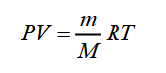In this formula:

• P is the gas pressure;
• V is the gas volume;
• m is the mass of the gas;
• M is the number of moles of the gas;
• R = 8,3145 J/(mol ∙ K) is the universal gas constant;
• T is the absolute gas temperature.

From the first formula, we get the expression for the molar mass of the gas: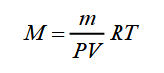Therefore, to calculate the molar mass of air, it is necessary to know the mass of the gas - m, the temperature - T, the gas pressure - P, and the volume it occupies.

If the measurement errors of P, V, and T in any experiment do not exceed 1%, then the determination of the gas mass is a difficult task. Complete gas removal from the vessel is almost impossible: even with the best modern engineering toolbox.

There is another way of determining M, in which it is not necessary to achieve complete removal of the gas from the vessel - it is enough to change its mass slightly. Let a vessel with volume V contain gas of mass m1 under pressure P1 and at temperature T. The equation of state for this gas will take the form: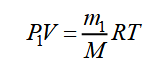We pump out part of the gas from the vessel without changing its temperature. After evacuation, the mass of gas in the vessel and partial pressures will decrease. Let us denote them, respectively, m2, P2, and rewrite the equation of state: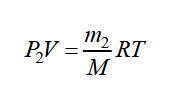From the equations, we get: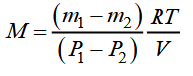This expression allows you to determine - M. If the change in the mass of the gas, in pressure, the temperature, the volume is known.

In this work, the investigated gas is air, known as a mixture of nitrogen, oxygen, carbon dioxide, argon, water vapor, and other gases. The above formula is also suitable for determining the M mixture of gases. In this case, the found value of M is a certain average or effective molar mass of the gas mixture.

## How To Theoretically Calculate The Molar Mass Of Air?

The molar mass of a mixture of gases (air), you can theoretically calculate. This method is suitable because the relative content and molar mass of each of the gases included in the mixture are known. The formula looks like this: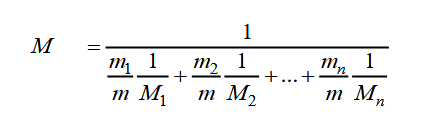In this formula:

• m1/m, m2/m are the relative fractions of the content of each gas;
• M1, M - molar masses of gases included in the mixture.

## How To Apply These Formulas In Practice?

In chemistry homework, teachers give advanced data, gas percentages, atomic weights, and different coefficients. Therefore, having all the data, you can apply the formulas. Let's take an example:

Task: Calculate the average molar mass of air has the following composition: 21% O2, 78% N2, 0,5% Ar, and 0,5% CO2.

Decision: Since the volumes of gases are proportional to their quantities (Avogadro's law), the average molar mass of a mixture can be expressed both in terms of volumes and in terms of quantities of substances:

Mav = (M1 · V1 + M2 · V2 + M3 · V3 +) / (V1 + V2 + V3 + ...) (1)
Mav = (M1 ν1 + M2 ν2 + M3 ν3 +) / (ν1 + ν2 + ν3 + ...) (2)

Take 1 mol of air, then v (O2) = 0,21 mol, v (N2) = 0,78 mol, v (Ar) = 0,005 mol and v (CO2) = 0,005 mol. Substituting these values into the formula, we get:

Mav (air) = (0,21· 32 + 0,78 · 28 + 0,005 · 40 + 0,005 · 44) / (0,21 + 0,78 + 0,005 + 0,005) = 29 g/mol.

That is why in numerous computational problems related to the relative density of gases in the air, the average molar mass of air is always assumed to be 29 g/mol.

Answer: Mav (air) = 29 g/mol.

## What Gases Are In The Air?

Atmospheric air is a mixture of gases. However, this is not a complete definition, and to expand it, we turn to history. In 1754, Scottish physicist and chemist Joseph Black, while heating white magnesia, discovered the release of "bound air." Having received CO2, Mr. Black made another significant discovery - the composition of the air, which was previously considered one substance, is not uniform.

Joseph Black showed the way to other scientists who, one after another, began to more and more decipher the composition of the atmosphere, calculate oxygen in the air, and other gases. The very definition was formed, which today sounds like this: air is a mixture of gases that form the Earth's atmosphere. The primary function of air is to make the planet suitable for breathing and the existence of living organisms.

Nitrogen gas occupies a large part of the air. However, the chemical composition of the remaining part is exciting and varied. In short, the list of essential elements is as follows:

1. Oxygen. The proportion of oxygen gas in the air fluctuates around 21% by volume and 23% by mass. Together with nitrogen, these two gases form 99% of all Earth's Air.
2. Argon. Argon is odorless, colorless, and tasteless. A significant biological role of this gas has not been identified, but it has a narcotic effect and is even considered doping.
3. Carbon dioxide. Carbon dioxide makes up the atmosphere of Venus and Mars. Its percentage in the Earth's air is much lower. At the same time, a considerable amount of carbon dioxide is contained in the ocean. In solid form, carbon dioxide is one of the best-known dry ice refrigerants.
4. Neon. Neon has a narcotic effect on a person at a certain pressure, but it is ideal gas used in the training of divers and other people working under total pressure.
5. Methane. Today, this gas, produced and used as a fuel and raw material in production, is not as widespread in the atmosphere, but it still escapes from the Earth.
6. Helium. This gas is not among the most important in terms of importance. It is difficult to determine the biological significance of this gas.
7. Krypton. Krypton is an inert gas three times heavier than air, chemically inert, extracted from the air, used in incandescent lamps, lasers and is still being actively studied.
8. Hydrogen. Hydrogen atoms in the air occupy 0,00005% by volume and 0,00008% by mass, but at the same time, they are the most common elements in the Universe.
9. Xenon. Nowadays, people cannot do without xenon: the production of powerful and pulsed light sources, diagnostics and anesthesia in medicine, spacecraft engines, rocket fuel.

### Air Density

What is the density of air? The density of a gas can be analytically determined by dividing its mass by the volume it occupies under given conditions (air pressure, absolute temperature, and humidity). You can also calculate its density using the ideal gas law: pV = nRT, where n is gas molecules, R is the gas constant. Thus, it is necessary to know the absolute pressure and temperature of the air and its gas constant and mole fraction. This equation calculates the dry density of air.

### Water Vapor

Due to the evaporation of water from the ocean's surface, moist air is always present in the air. The amount of humid air depends on the air temperature, the presence and speed of the wind, the nature of the relief, the vegetation cover, and the soil's color.

According to Dalton's law, air pressure containing water vapor is the sum of the pressure of dry air and the pressure of water vapor. Atmospheric pressure is: Pa = Pda + Pp.

The humidity of the air can be judged either by the magnitude of the pressure produced by the steam. The amount of water vapor contained in 1 m3 of air is called absolute humidity. The ratio of water vapor density at a given temperature to the saturated vapor density at the same temperature is called relative humidity.

## Air Properties

Air has many properties, and you need to know about these:

1. The air is transparent, colorless, odorless, and does not conduct heat well.
2. Air conducts sunlight well.
3. Air takes up space in the surrounding world.
4. Air can be compressed.
5. Air is elastic.
6. Air expands when heated; when cooled, air contracts.
Warm air is lighter than cold air and tends upward.

User ratings:

User ratings is 4.6 stars.Angelina Grin

Creative Writer and Blog Editor

Despite my relatively young age, I am a professional writer with more than 14 years of experience. I studied journalism at the university, worked for media and digital agencies, and organized several events for ed-tech companies. Yet for the last 6 years, I've worked mainly in marketing. Here, at Studybay, my objective is to make sure all our texts are clear, informative, and engaging.